（1）策略层面，根据系统和业务特性设计规则，比如说对长尾的 item 有特定的扶持，强行让这些 item 能触达到更多的用户
（2）模型层面，核心思想就是让模型能更好地学习到 long tail item 的 representation，因为这个问题的根本原因就是 long tail item 的样本过少，进而导致模型学习的不好；而具体的手段比较多，这部分会在后面详细介绍。

## Dual Transfer Learning Framework

paper 名字中提到的 dual transfer learning，分别是 model-level 和 item-level 的 transfer learning，简单来说，前者让样本少的模型(few-shot model)的参数尽可能往样本多的模型(many-shot model)的参数靠拢(这里根据样本量分为 2 个模型来建模)，后者则是让 long tail item 的 representation 与 head item 的尽可能接近，这里的 representation 其实就是上面提到的 few-shot model 和 many-shot model 吐出来的 embedding，因此 paper 提出的总体框架如下如所示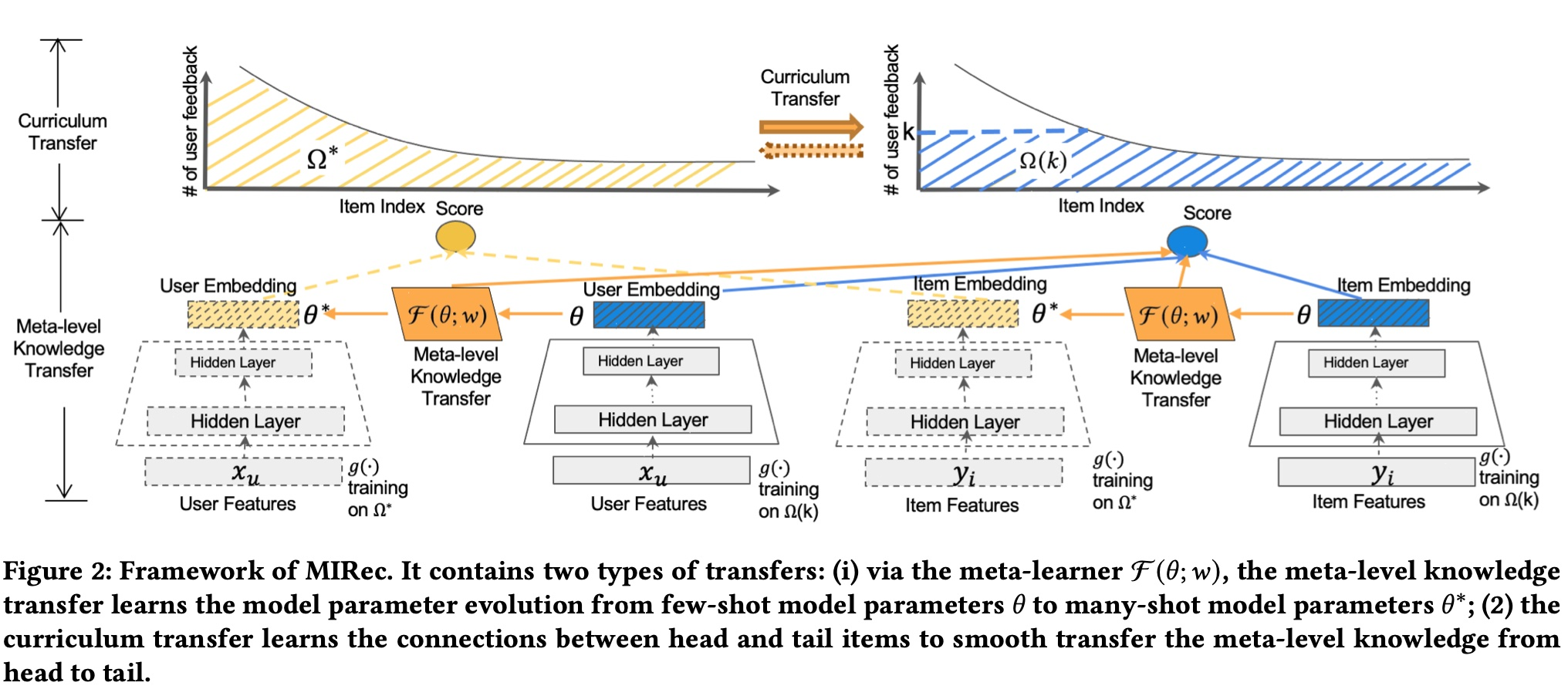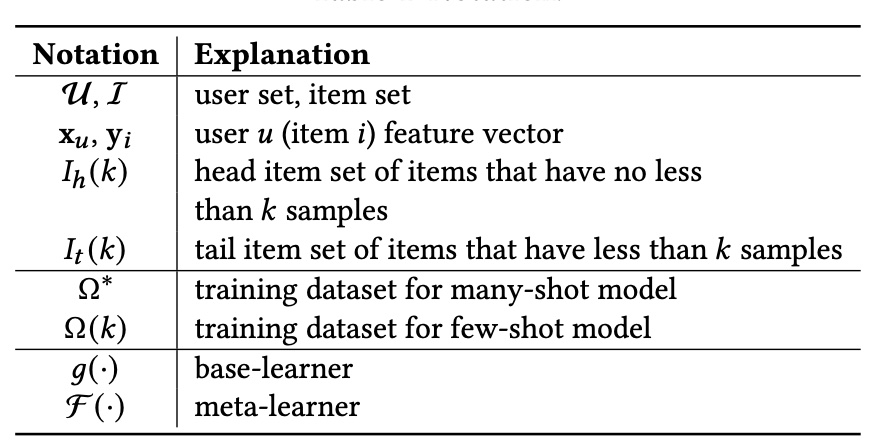### model-level

parameter，监督信号是输出的 parameter 要与 many-shot model 的 parameter 接近，最终作用方式是在 loss 上

base learner 的 loss 是常规的 softmax loss，$r(u,i)$ 取值为 1/0 表示是否有 feedback(如 click 等)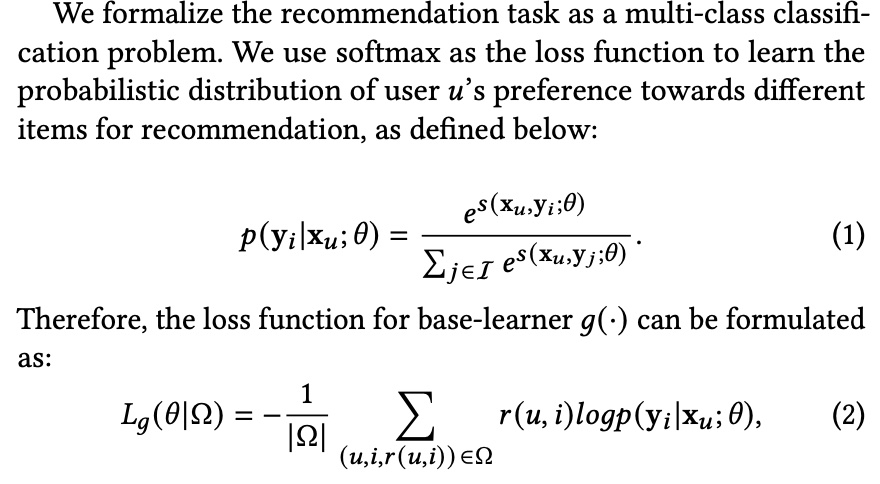meta learner $\mathcal{F}$ 则是采用了 mse 的 loss，并且作为一个 regularization 项加到 few-shot model 原始的 loss 上，则最终 few-shot model 的 loss 如下公式(5)所示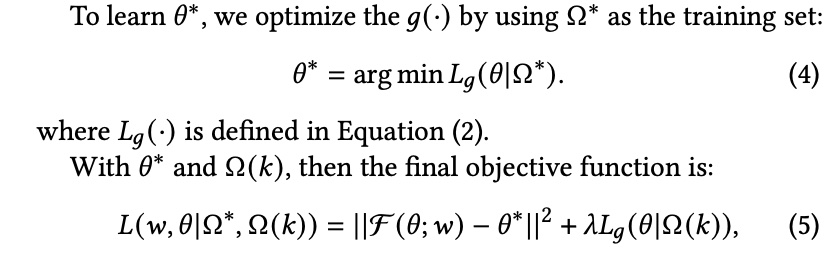meta learner $\mathcal{F}$ 具体的结构有很多种，这里采用的是简单的 fully connected layer

### item-level

Curriculum learning (CL) is a training strategy that trains a machine learning model from easier data to harder data, which imitates the meaningful learning order in human curricula. As an easy-to-use plug-in, the CL strategy has demonstrated its power in improving the generalization capacity and convergence rate of various models in a wide range of scenarios such as computer vision and natural language processing etc.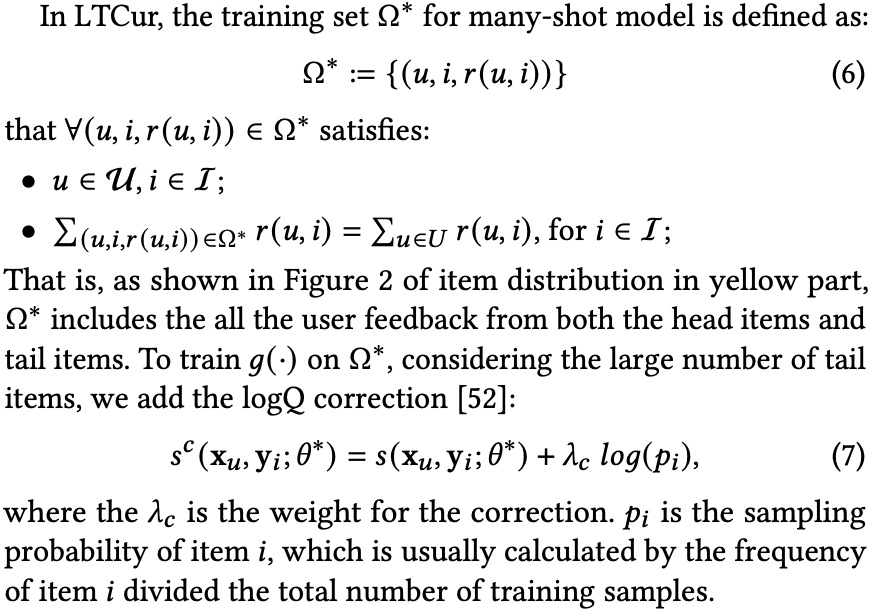$\Omega(k)$ 的构造方法如下，这部分样本包含 2 部分 item，一部分是 $I_{h}(k)$ 中那些刚好有 k 个sample 的 item，另一部分则是 $I_{t}(k)$ 中的全部 sample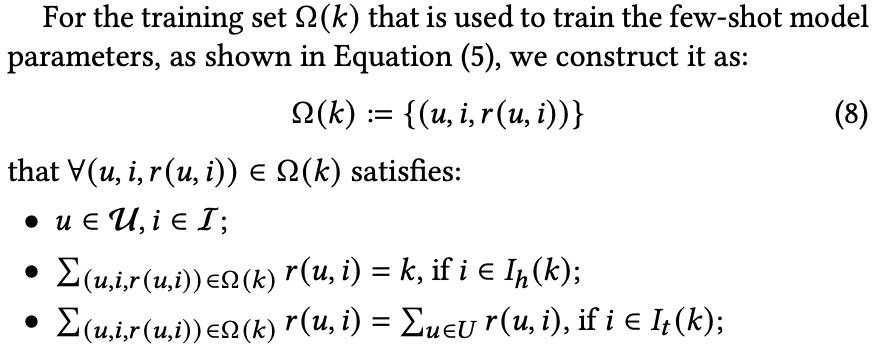paper 里称这么做主要有以下 2 个原因，但是笔者觉得核心还是把 long tail item 的样本单独出来，不至于被 head item dominate

(1) tail items are fully trained in both the many-shot model and few-shot model to ensure the high quality of the learned item representations in both many-shot and few-shot models
(2）In the few-shot model training, the distribution of tail items relatively keeps the same as the original distribution. It can alleviate the bias among tail items that brings by the new distribution

### training & serving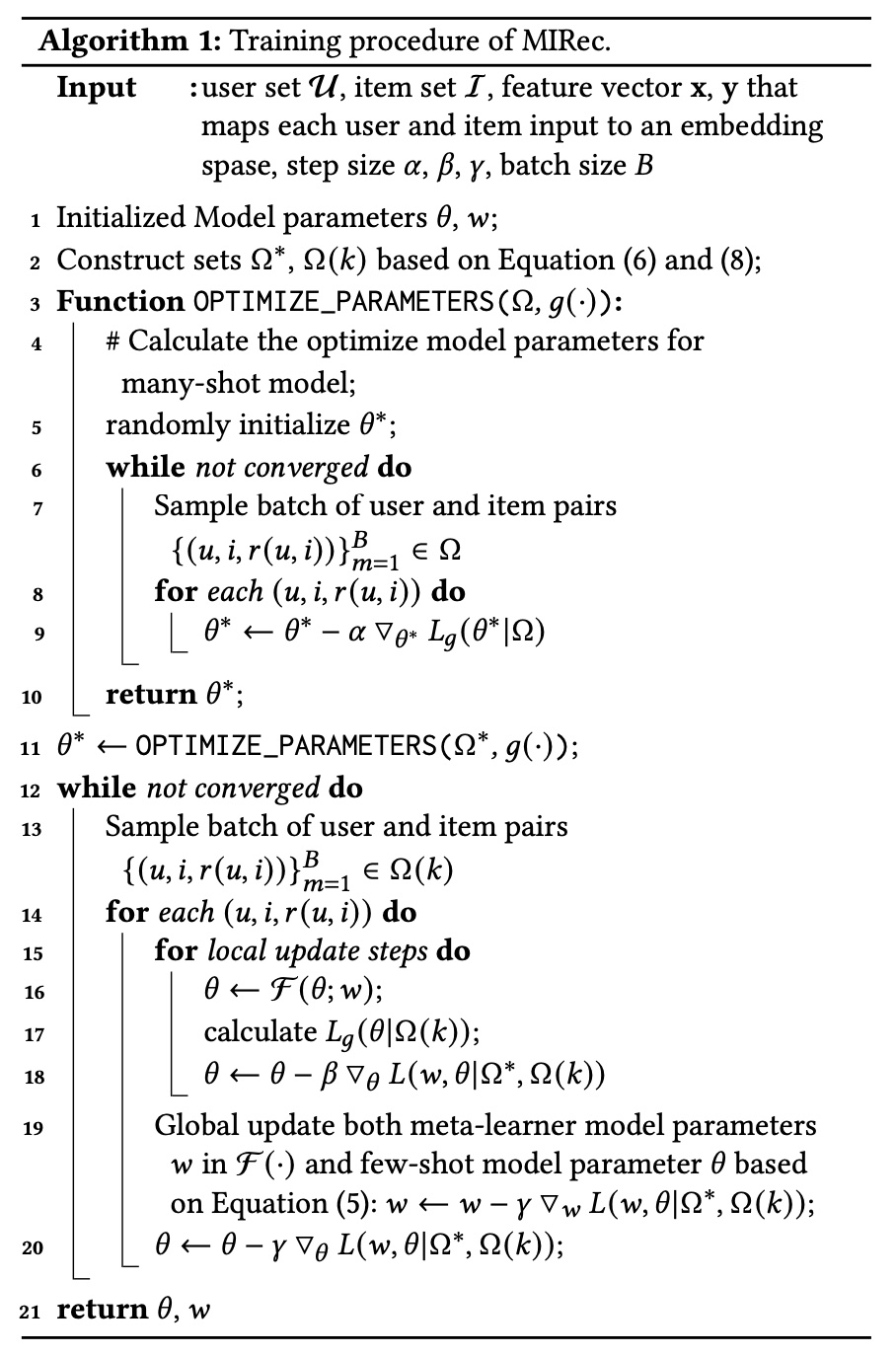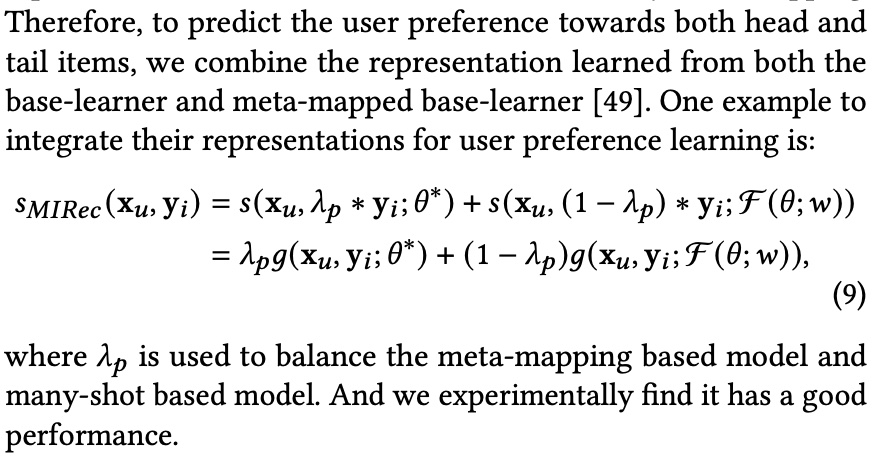### experiment

paper 里的实验用了 2 个公开的数据集，MovieLens1M 和 Bookcrossing，采用的评估指标是Hit Ratio at top K (HR@K) 和 NDCG at top K (NDCG@K) ，这里的 HR@K 其实就是召回率

paper 里的实验着重回答了以下四个问题

RQ1: How well does the dual transfer learning framework MIRec perform compared to the state-of-the-art methods?
RQ2: How do different components (meta-learning and curriculum learning) of MIRec perform individually? Could they complement each other?
RQ3: How does our proposed curriculum learning strategy compare with the alternatives?
RQ4: Besides downstream task performance, are we actually learning better representations for tail items? Could we see the differences visually?

(1) Compared to the tail item loss in different curriculums (column 3), our proposed curriculum can bring a two-stage decent for both the training and validation loss
(2) When the model is trained based on only head/tail items, the validation performance for the other part of items decreases. The different changes of head and tail loss indicate the large variations between head and tail items
(3) It is easily to get validation loss increases if the model is trained purely based on head/tail items, as shown in first column of the first two rows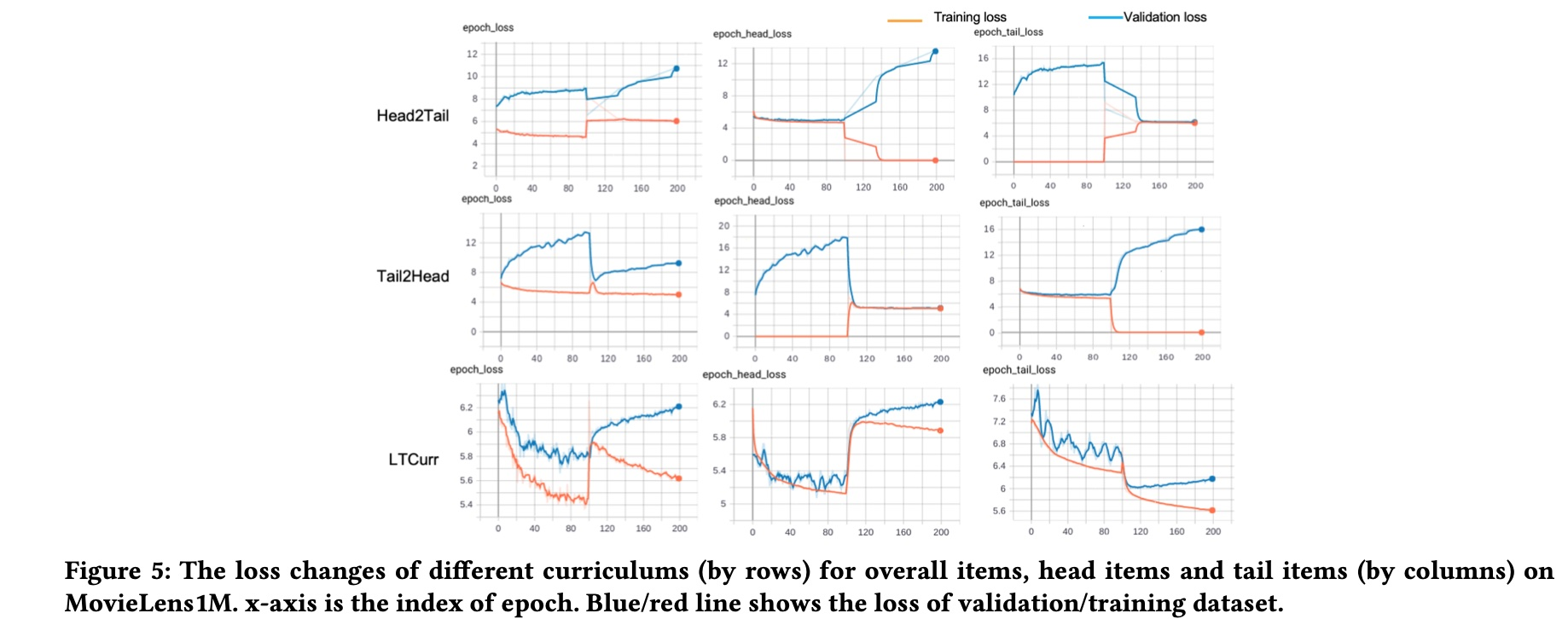## Self-supervised Learning Framework

The framework is designed to tackle the label sparsity problem by learning better latent relationship of item features. Specifically, SSL improves item representation learning as well as serving as additional regularization to improve generalization. Furthermore, we propose a novel data augmentation method that utilizes feature correlations within the proposed framework.

### SSL Framework

paper 提出的总体的 framework 如下图所示，基本符号的含义都在图片下方的注释里，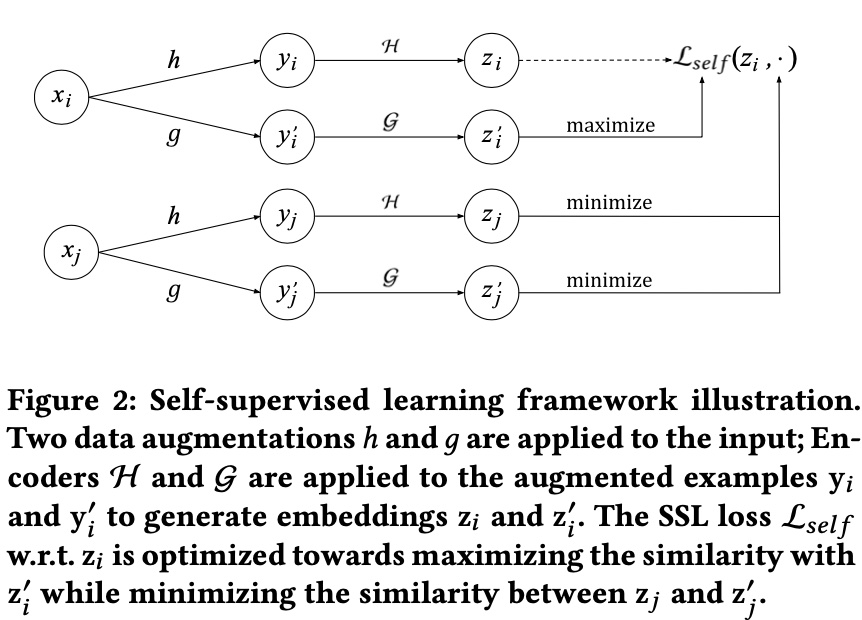$$\mathcal{L}_{self}(x_i) = -\log \frac{\exp(s(z_i, z_i^{‘})/\tau)}{\sum_{j=1}^{N}\exp(s(z_i, z_j^{‘})/\tau)}$$

$$\mathcal{L}_{self}(\lbrace x_i \rbrace; \mathcal{H}, \mathcal{G}) = - \frac{1}{N} \sum_{i=1}^{N} \log \frac{\exp(s(z_i, z_i^{‘})/\tau)}{\sum_{j=1}^{N}\exp(s(z_i, z_j^{‘})/\tau)}$$

$$-\frac{1}{N} \sum_{i=1}^{N} \log \frac{\exp(1/\tau)}{ \exp(1/\tau) + \sum_{j \ne i}\exp(s(z_i, z_j^{‘})/\tau)}$$

### two-stage data augmentation

paper 里采用的方法则是 mask, 这里借鉴了 bert 的思想，但是这里没有 sequence 的概念，因此这里 mask 掉的是 item feature，实际使用的是一个 two-stage 的方法，即 masking + dropout, 两个方法主要操作如下

Masking. Apply a masking pattern on the set of item features. We use a default embedding in the input layer to represent the features that are masked.
Dropout.For categorical features with multiple values,we drop out each value with a certain probability. It further reduces input information and increase the hardness of SSL task.

### training & serving

$$\mathcal{L}_{main} = -\frac{1}{N} \sum_{i=1}^{N} \log \frac{\exp(s(q_i, x_i)/\tau)}{\sum_{j=1}^{N}\exp(s(q_i, x_j)/\tau)}$$

$$\mathcal{L} = \mathcal{L}_{main} + \alpha \mathcal{L}_{self}$$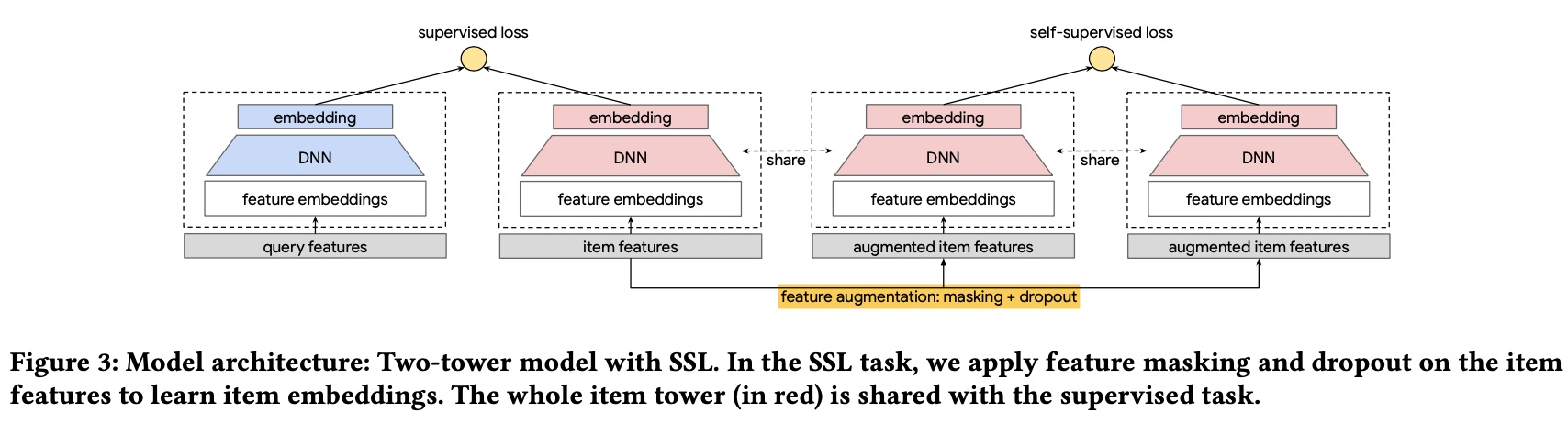### experiment

RQ1: Does the proposed SSL Framework improve deep models for recommendations?

RQ2:SSL is designed to improve primary supervised task through introduced SSL task on unlabeled examples. What is the impact of the amount of training data on the improvement from SSL?

RQ3: How do the SSL parameters, i.e., loss multiplier $\alpha$ and dropout rate in data augmentation, affect model quality?

RQ4: How does RFM perform compared to CFM? What is the benefit of leveraging feature correlations in data augmentation?

RQ1，通过与其他 3 个 baseline 进行了比较，采用了 2 个公开数据，评估指标是 MAP@10/50 和 Recall@10/50, 跟前一篇 paper 一样，做了总体 item、head item 和 tail item 各自的评估

RQ2 主要回答数据量对 SSL 的影响，结论是数据量越多，效果越好，感觉这个结论比较符合直觉，不知道 paper 为什么要单独拎出来说

RQ3 主要回答 $\alpha$ 大小对效果的影响，paper 里对比 spread-out regularization loss 和 paper 提出的 self supervised loss，结论是取相同的值时均是 self supervised loss 效果更好，关于 spread-out regularization loss 可参考这篇 paper，也是一种 constrasive loss，但是没有 data augmentation

RQ4 主要回答随机 mask 的方法(RFM) 和基于相关性 mask 的方法(CFM)哪种效果更好，结论是CFM在四项指标上效果均优于 RFM